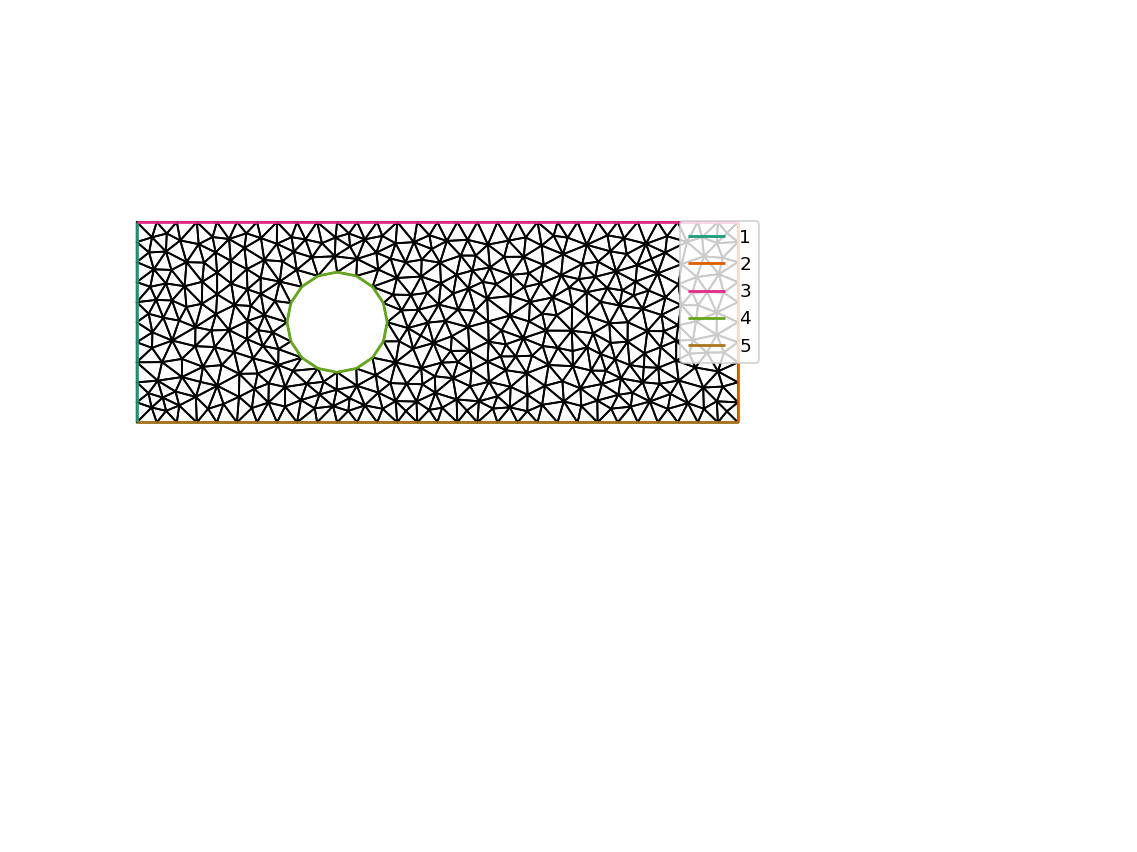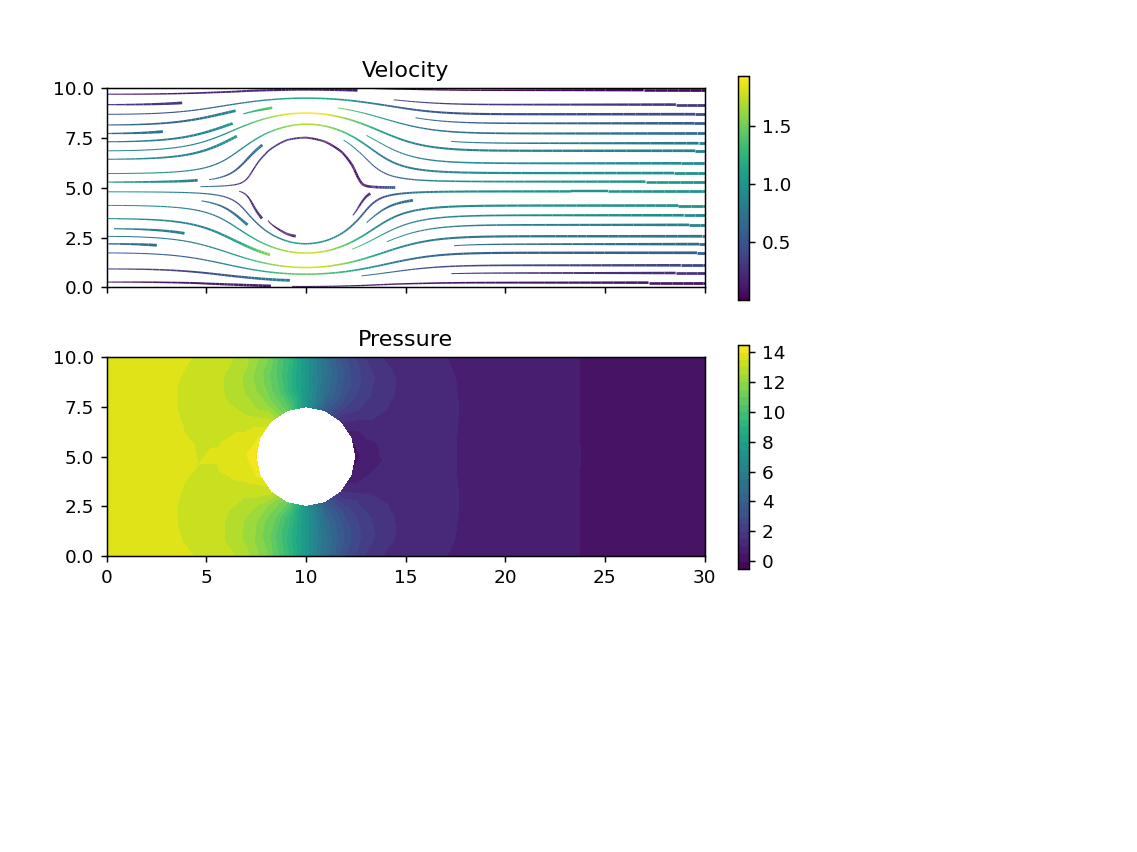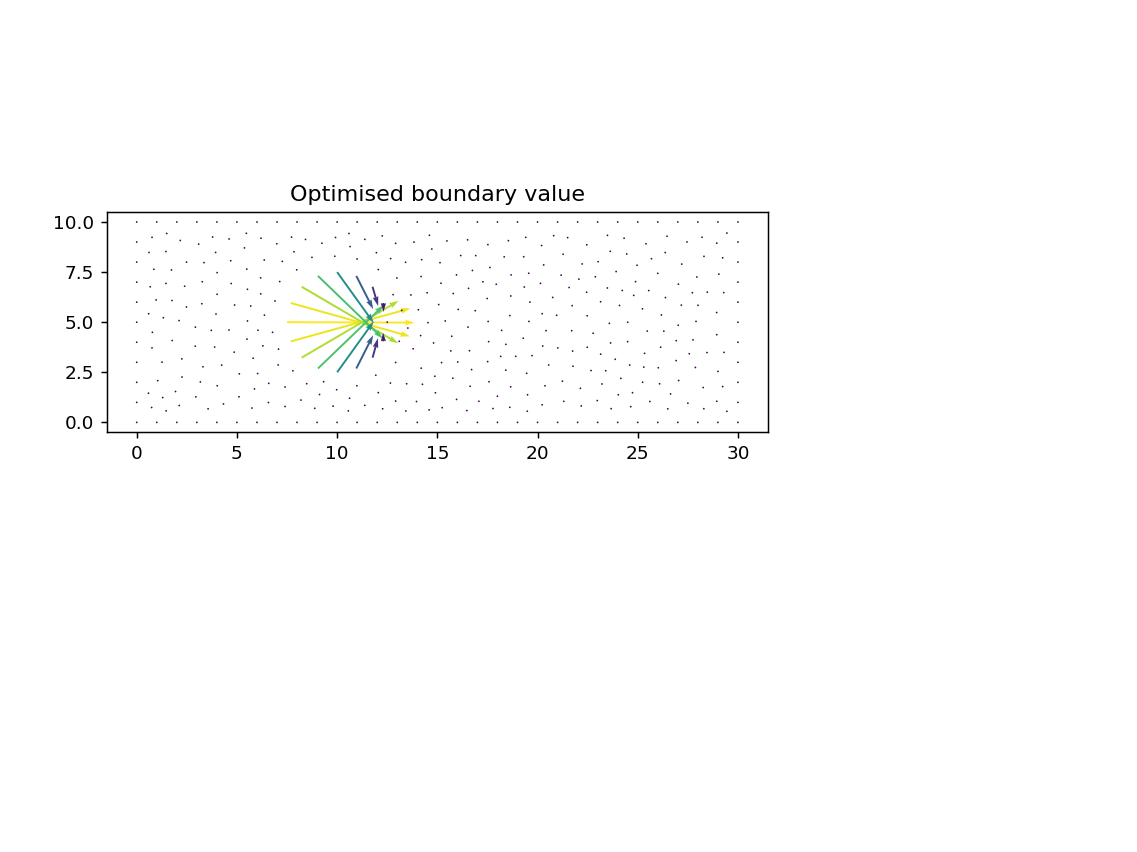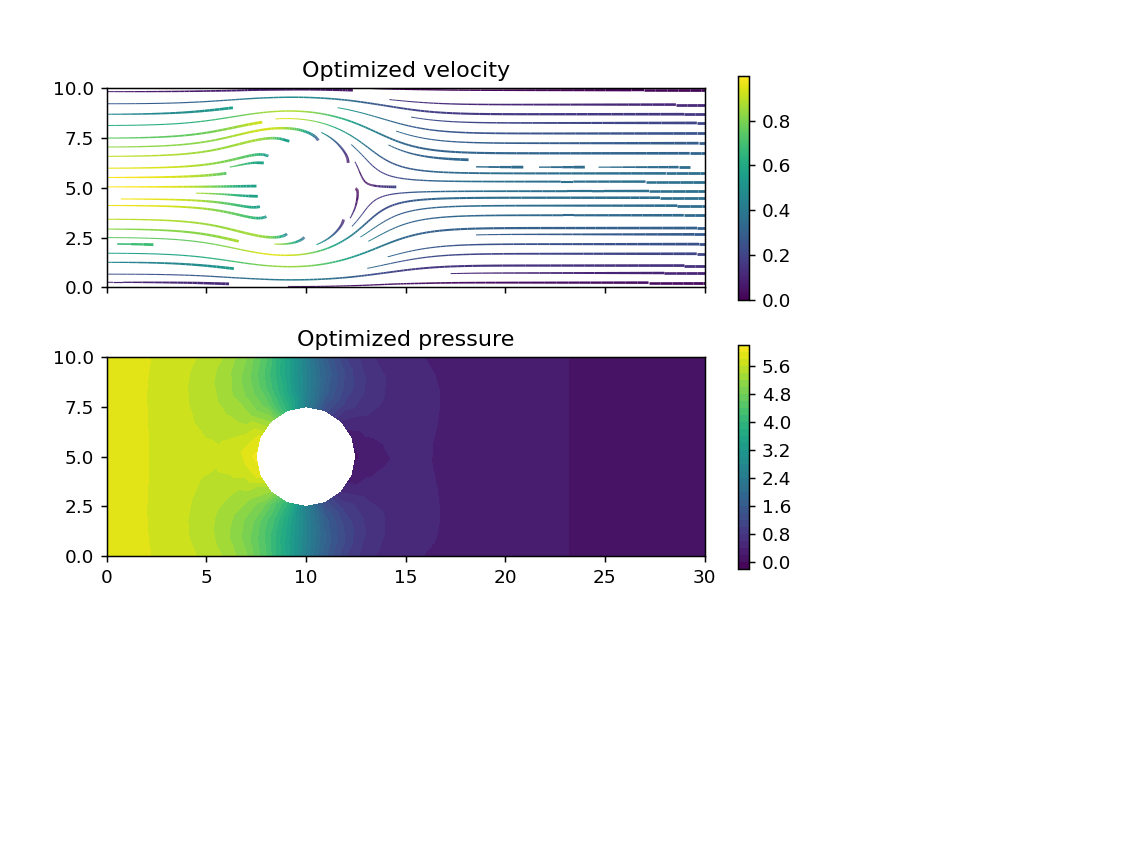# Optimisation with firedrake-adjoint¶

This example is modified from the equivalent dolfin-adjoint demo

In this example, we will look at how to use firedrake-adjoint to optimise for strong (Dirichlet) conditions in a steady problem. firedrake-adjoint is a thin compatibility layer for the dolfin-adjoint package, a python package to automatically derive the discrete adjoint and tangent linear models of forward problems written using Firedrake.

## Installing necessary dependencies¶

For the minimisation, we will need scipy, which is installed with

pip install scipy


In the activated firedrake virtualenv.

As usual, we begin with some notebook magic (so that plots appear nicely) and importing Firedrake.

In :
%matplotlib notebook
import matplotlib.pyplot as plt
from firedrake import *


The next step is new, we now import the firedrake_adjoint package, which overrides much of the Firedrake interface so that an annotated tape for adjoint calculations can be built automatically.

In :
from firedrake_adjoint import *


## Problem setup¶

Now we will set up the problem. We consider minimising the compliance:

$$\min_{g, u, p} \ \frac{1}{2}\int_{\Omega} \nabla u \cdot \nabla u\,\text{d}x + \frac{\alpha}{2} \int_{\Gamma_{\textrm{in}}} g^2\,\text{d}s$$

subject to the Stokes equations $$\begin{split}-\nu \Delta u + \nabla p &= 0 \quad \text{in \Omega} \\ \nabla \cdot u &= 0 \quad \text{in \Omega} \end{split}$$

and Dirichlet conditions

$$\begin{split} u &= g \quad \text{on \Gamma_\text{circ}} \\ u &= f \quad \text{on \Gamma_\text{in}} \\ u &= 0 \quad \text{on \Gamma_\text{top} \cup \Gamma_\text{bottom}} \\ p &= 0 \quad \text{on \Gamma_\text{out}}. \\ \end{split}$$

Here, $u$ and $p$ are unknown velocity and pressure, $f$ is a prescribed inflow, $g$ is the control variable that we will optimise for and $\alpha$ is a regularisation parameter. This corresponds physically to minimising the loss of energy as heat by controlling the in/outflow on $\Gamma_\text{circ}$. The regularisation parameter penalises too many non-zero control values.

This problem setup requires a mesh that is more complex than the built in ones Firedrake provides. Instead, it was created with Gmsh. It is loaded by using the Mesh constructor, passing the filename of the mesh in question.

In :
mesh = Mesh("stokes-control.msh")


Now we'll take a look at the mesh. Since we will need to know which mesh markers correspond to which parts of the boundary, we've added a legend to the plot. Normally you will know this because you told your mesh generator how to mark the boundaries.

In :
# NBVAL_IGNORE_OUTPUT
fig, axes = plt.subplots()
triplot(mesh, axes=axes)
axes.axis("off")
axes.set_aspect("equal")
axes.legend(loc="upper right");## The forward problem¶

The forward problem should be familiar by now, we create a mixed function space for $u$ and $p$ and set up trial and test functions. We specify a parabolic velocity at the inflow, and no-slip (zero velocity) conditions on the side walls. The zero-pressure outflow condition is enforced weakly.

Our variational formulation for Stokes reads as follows. Find $(u, p) \in V\times Q$ such that:

\begin{align} \int_\Omega \nu \nabla u : \nabla v\,\text{d}x - \int_\Omega p \nabla \cdot v\,\text{d}x - \int_{\Gamma_{\text{circ}}} \nu (\nabla u \cdot n) \cdot v\,\text{d}s &= 0 \quad \forall v \in V\\ - \int_\Omega q \nabla \cdot u\,\text{d}x &= 0 \quad \forall q \in Q.\\ u &= 0 \quad \text{on \Gamma_{\text{top}} \cup \Gamma_{\text{bottom}}}\\ u &= \left[\frac{y(10 - y)}{25}, 0\right]^T \quad \text{on \Gamma_{\text{in}}}\\ u &= g \quad \text{on \Gamma_{\text{circ}}}\\ \frac{\text{d}p}{\text{d}n} &= 0 \quad \text{on \Gamma_{\text{out}}} \end{align}
In :
V = VectorFunctionSpace(mesh, "CG", 2)
Q = FunctionSpace(mesh, "CG", 1)
W = V*Q

v, q = TestFunctions(W)
u, p = TrialFunctions(W)

nu = Constant(1)     # Viscosity coefficient

x, y = SpatialCoordinate(mesh)
u_inflow = as_vector([y*(10-y)/25.0, 0])

noslip = DirichletBC(W.sub(0), (0, 0), (3, 5))
inflow = DirichletBC(W.sub(0), interpolate(u_inflow, V), 1)
static_bcs = [inflow, noslip]


The boundary value, $g$, on the circle will be our control variable. To do this, we define a Function which will hold the boundary values, we then build a DirichletBC object as normal.

In :
g = Function(V, name="Control")
controlled_bcs = [DirichletBC(W.sub(0), g, 4)]
bcs = static_bcs + controlled_bcs


Now we define the bilinear and linear forms.

In :
a = nu*inner(grad(u), grad(v))*dx - inner(p, div(v))*dx - inner(q, div(u))*dx
L = Constant(0)*q*dx


Now let's solve the forward problem so that firedrake-adjoint annotates it. We'll also take a look at the solution.

In :
w = Function(W)
solve(a == L, w, bcs=bcs, solver_parameters={"pc_type": "lu", "mat_type": "aij",
"pc_factor_shift_type": "inblocks"})

In :
# NBVAL_IGNORE_OUTPUT
u_init, p_init = w.split()

fig, axes = plt.subplots(nrows=2, sharex=True, sharey=True)
streamlines = streamplot(u_init, resolution=1/3, seed=0, axes=axes)
fig.colorbar(streamlines, ax=axes, fraction=0.046)
axes.set_aspect("equal")
axes.set_title("Velocity")

contours = tricontourf(p_init, 30, axes=axes)
fig.colorbar(contours, ax=axes, fraction=0.046)
axes.set_aspect("equal")
axes.set_title("Pressure");## The optimisation problem¶

Now we come to the optimisation problem. We first define the functional we wish to minimise, this is done by assembling a form that produces a number. Then we specify a control variable, and produce a "reduced" functional (the evaluation of the functional at a given control value). We then minimise the functional, producing an optimised control value. See the dolfin-adjoint documentation for more details on reduced functionals.

In :
u, p = split(w)
alpha = Constant(10)

m = Control(g)
Jhat = ReducedFunctional(J, m)

In :
g_opt = minimize(Jhat)


Now let's take a look at the optimised control. The initial boundary condition on the circle was no slip. We see now that the optimised boundary condition has a significant outflow in the hole.

In :
# NBVAL_IGNORE_OUTPUT
fig, axes = plt.subplots()
arrows = quiver(g_opt, axes=axes, scale=3)
axes.set_aspect("equal")
axes.set_title("Optimised boundary value");Let's compare the difference in the initial and final values of the functional. This is done by calling the ReducedFunctional object with the control at the requested value.

In :
print("Jhat(g) = %.8g\nJhat(g_opt) = %.8g" % (Jhat(g), Jhat(g_opt)))

Jhat(g) = 46.078212
Jhat(g_opt) = 19.896719


To see the optimised flow field, we solve the same problem again, only with the new (optimised) value for the boundary data on $\Gamma_\text{circ}$. This time we're not interested in annotating the solve, so we tell firedrake-adjoint to ignore it by passing annotate=False.

In :
g.assign(g_opt)
w_opt = Function(W)
# Use context manager
solve(a == L, w_opt, bcs=bcs, solver_parameters={"pc_type": "lu", "mat_type": "aij",
"pc_factor_shift_type": "inblocks"},
annotate=False)

In :
# NBVAL_IGNORE_OUTPUT
u_opt, p_opt = w_opt.split()

fig, axes = plt.subplots(nrows=2, sharex=True, sharey=True)
streamlines = streamplot(u_opt, resolution=1/3, seed=0, axes=axes)
fig.colorbar(streamlines, ax=axes, fraction=0.046)
axes.set_aspect("equal")
axes.set_title("Optimized velocity")

contours = tricontourf(p_opt, 30, axes=axes)
fig.colorbar(contours, ax=axes, fraction=0.046)
axes.set_aspect("equal")
axes.set_title("Optimized pressure");Now that we are done optimising this problem, and so that the recorded annotations do not leak into the exercise, we tell firedrake-adjoint to forget all of the entries on its tape.

In :
tape = get_working_tape()
tape.clear_tape()


## Exercise:¶

Go back to the start of the notebook and consider a different inflow velocity. For example, try $$u = \left[\frac{y^2(10 - y)}{125}, 0\right]^T \quad \text{on} \quad \Gamma_{\text{in}}.$$

How does it affect the solution before and after optimisation?